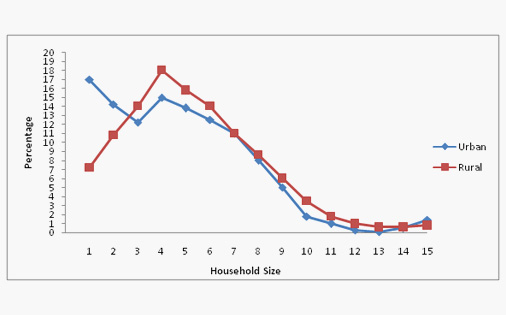304 North Cardinal St.
Dorchester Center, MA 02124

# Data Interpretation Practice Questions

-

The following is the Data Interpretation Practice Questions section. We advise you to go through the Data Interpretation Practice Questions once after you are done with the section on data interpretation. Let us see more.

There are four type of Data interpretation Question that may be asked in Bank/ IBPS Bank :

1. Table
2. Line
3. Bar
4. Pie

Here below We have given example question different data interpretation questions. We recommend you to go through this carefully and practice many data interpretation questions.

## Table Data Interpretation Questions :

Table : DIRECTIONS for questions 1 to 3: The following table gives the data about big 12 software exporters in India from 1997-98 to 1999-2000 (Revenue in Rs. crore).

Questions 1: What is the lowest rate of growth of 1998-99 to 1999-2000 for any of the companies given in the chart?

A. 0%

B. 12.7%

-

C. 19.1%

D. 10.1%

E. 5.3%

Explanation: Lowest growth has been for HCL: from 862 to 908, about 5%. Hence option E

Question 2: Which of the following statement is true about the share of exports of Tata Consultancy Services in the total exports of the 12 companies?A. It has almost doubled in 1999 – 00 over 1997 – 98.B. It has been steadily increasingC. It is more or less constant.D. It has dropped by about 10 %.E. None of these.

A. It has almost doubled in 1999 – 00 over 1997 – 98.

B. It has been steadily increasing

C. It is more or less constant.

D. It has dropped by about 10 %.

E. None of these.

Explanation: Share in 1997-98: 33%.
In 1999-2000, the share is 1820/7618 = 23%, so it has dropped by almost 10%.

Question 3 : If total software exports in 2000 – 2001 were expected to be Rs 10,000 crore and the growth of Infosys is the same as it was in 1999 – 2000, what would have been Infosys’ share of exports of these 12 companies in 2000 – 2001?

A. 15%

B. 19%

C. 21%

D. 23%

E. None of these

Explanation: Growth of Infosys has been almost 74%.
Increase 869 by 74 % and we get about 1,500 which is 15% of 10,000.

## Graph Based Data Interpretation Questions

DIRECTIONS for questions 6 to 10: The percentage distribution of households by household size and the average sizes of household in.

• All India Rural and Urban areas.
• Urban areas classified as per population size are presented below.Question 1: In rural areas, which one of the following sizes of the households is the highest in number?

A. 15

B. 2

C. 3

D. 4

E. Can’t be determined

Explanation: From the graph, we see that the line for rural peaks at about 4-5.
Hence the best answer is (4).

Question 2: In urban areas, among the households of different sizes, what is the percentage of households of size 5 or less?

A. 13

B. 72

C. 36

D. 87

E. Can’t be determined

Explanation: Total up the number of households ≤ 5 are 17 + 14 + 12 + 15 + 14 = 72.

Question 4 In rural areas, per 100 households, there were 31 households of size:

A. 3 or above

B. 3, 4 and 5

C. 3 or less

D. 4 or less

E. 5 or more

Explanation: Total up the values for rural area for the size 3 or less to get the answer.

Question 5: Which one of the following statements is true?

A.On the average there are more persons per family in urban areas than in rural areas.

B.In rural areas, 35 per cent of the households are of the size 7 and above.

C.In urban areas, the average size of the household is the least for towns.

D.In urban areas, there are 460 persons on an average per 100 households.

E.None of these

Explanation: Only statement (b) can be inferred from the given graphs.

## Pie Chart Based Data Interpretation Questions

The following pie-chart shows the percentage distribution of the expenditure incurred in publishing a book. Study the pie-chart and the answer the questions based on it.

Various Expenditures (in percentage) Incurred in Publishing a Book

Question 1: If for a certain quantity of books, the publisher has to pay Rs. 30,600 as printing cost, then what will be amount of royalty to be paid for these books?

A. Rs 19450

B. Rs 19110

C. Rs. 22,950

D. Rs. 23,910

C

Then, 20 : 15 = 30600 : r

Question 2:

A. Rs. 187.50

B. Rs. 188.3

C. 122.4

D.123.3

(Qs. 1-5) study the following table and answer the questions given below it.

Production of sugar by six major production units of India in Million Tonnes:

1. In which month unit B has a contribution of approximately 15% in the total sugar production?

a) August         b) June           c) July               d) April

2. Which of the following units shows continuous increase in production of sugar over months?

a) A           b) B          c) C            d) D

3. In the case of Unit E, in which of the following pairs of months the production of sugar was equal?

a) April & June          b) June & July          c) July & August              d) April & May

4. In the month of June, how many units have a share of more than 25% of the total production of sugar?

a) one          b) Three              c) Two                d) Four

5. What was approximate percentage decrease in sugar production of unit B in June as compared to April?

a) 8 %          b) 10 %             c) 15 %             d) 18 %